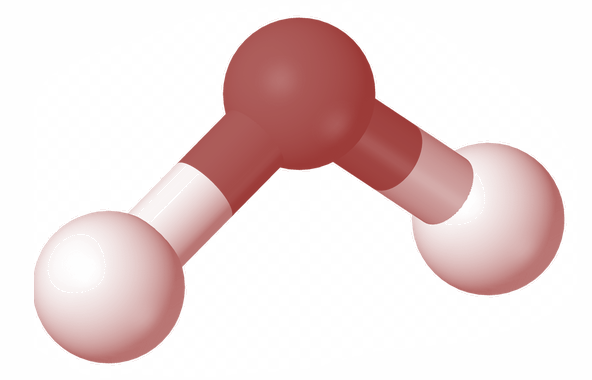QuestionAnswers

# Degree of freedom of a triatomic gas is? (Consider moderate temperature)A. 6B. 4C. 2D. 8

Hint: Degree of freedom is the number of independent motions a particle can undergo. To find out the degree of freedom we have to find the number of independent motions possible for that particular molecule. Here we have a triatomic gas and in order to find its degree of freedom, we check its possible motions in x, y and z directions.

We have to find the Degree of freedom of a triatomic molecule. A triatomic gas molecule has 3 atoms in it.Consider a triatomic gas molecule as shown in the figure above.
We now consider the possible movements of this molecule in the x, y and z axis.
Here this triatomic gas can have a translatory motion along the x, y, and z axis. I.e. triatomic molecules can move along x direction, y direction and z direction.
Hence the translatory degree of freedom of this molecule is 3
Now let us consider the rotational degree of freedom of this molecule.
For that we place two atoms of the molecule on the x axis. Then it can rotate about y axis and z axis. It also has a significant rotation about x axis because here the third atom has a moment of inertia about x axis even if the other two atoms do not have the inertia.
And thus the rotational degree of freedom of this molecule is also three.
Hence the degree of freedom= Translatory degree of freedom + Rotational degree of freedom
=3+3=6
Therefore at moderate temperature the degree of freedom of a triatomic gas equals to 6.

So, the correct answer is “Option A”.

Note: We can also find the degree of freedom by using the general expression
Degree of freedom, $\text{DF=3N-n}$ where N is the total number of particles and n is holonomic constraints.
We have a triatomic molecule. And hence the number of particles, $\text{N=3}$. And since the separation between 3 atoms is fixed, the number of holonomic constraints, $\text{n=3}$.
Therefore we have,
\begin{align} & \text{DF=3 }\!\!\times\!\!\text{ 3 - 3} \\ & \text{DF=9 - 3} \\ & \text{DF=6} \\ \end{align}
Thus the degree of freedom of a triatomic molecule is 6.